ValueBet Real Yield (ROI) • finderBet

# ValueBet Real Yield

## Valuebet: FinderBet annuity VS Real annuity

### ValueBet: Example chart

Below is an example of a published graph, with an explanation of the individual parts: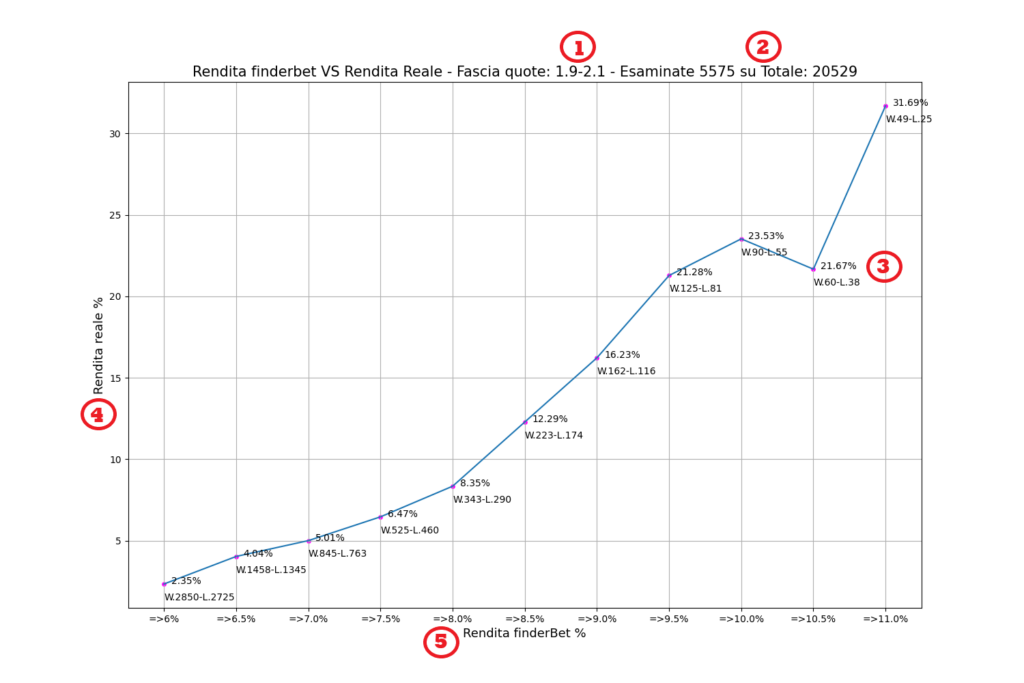1. Odds range: The example takes into consideration the odds between 1.8 and 2.2. In the law of large numbers, deriving from the medium/long-term income game, the number of lost/won bets balances out and in the case of number of wins = number of losses the probability must be at least 50% in favor, so as to ensure balance the budget and confidently aspire to profit. It follows that in all the graphs shown, the quota bands straddling quota 2 are taken into consideration, such as: 1.9-2.1 / 1.8-2.2 / 1.7-2.3 / 1.6-2.4 / 1.5-2.5. From the study conducted, a further widening of the quota bands leads to an unprofitable dispersion of capital.
2. Examine …. on Total: the most important data is represented by the total of the odds that have been analysed, both won and lost for a given odds range, a data that will be constantly updated in the time, to re-determine the real value bet income based on the total volume of bets.
3. ROI% – Lose & Win: This is the REAL percentage of return from ValueBet finderBet (ROI). We have added the number of games won (W) and the number of games lost (L) in the second line, so as to have the total W+L of the odds that are playable for a given range.
4. Real Income %: Scale of the real income determined by the ValueBet and this is what determines the profit over time (ROI)
5. FinnderBet return %: It is the percentage shown on the ValueBet page at the beginning of each line. This is a parameter to determine the quality of the ValueBet, the higher it is, the more it determines a deviation in the odds compared to the others analyzed. The => is shown in the graphs, this means that all those odds that have a % higher than , determining a distinction of the odds not only by bands, but also by goodness, are included in the statistics. In this case the study determined that percentages below <6% do not result in a profit over time.

Taking inspiration from the example and reporting in the description we have:

• Total odds analyzed for statistical purposes = 5575 out of a Total = 20529
• which belong to the 1.9-2.1
• quota range

• which for example for =>10.5% there is a total of wins W.60 + lossesL.38 = Total played 98

Reporting the data from the graph:

FinderBet percentage =>10.5% VS Real percentage 21.67% – Playable Valuebets= won W.60 + lost L.38 = 98

### ValueBet: Statistics, January 2022 to the present.

Below is the data from the statistical study conducted on the real income of the ValueBet finderBet, a study that we will constantly update over time: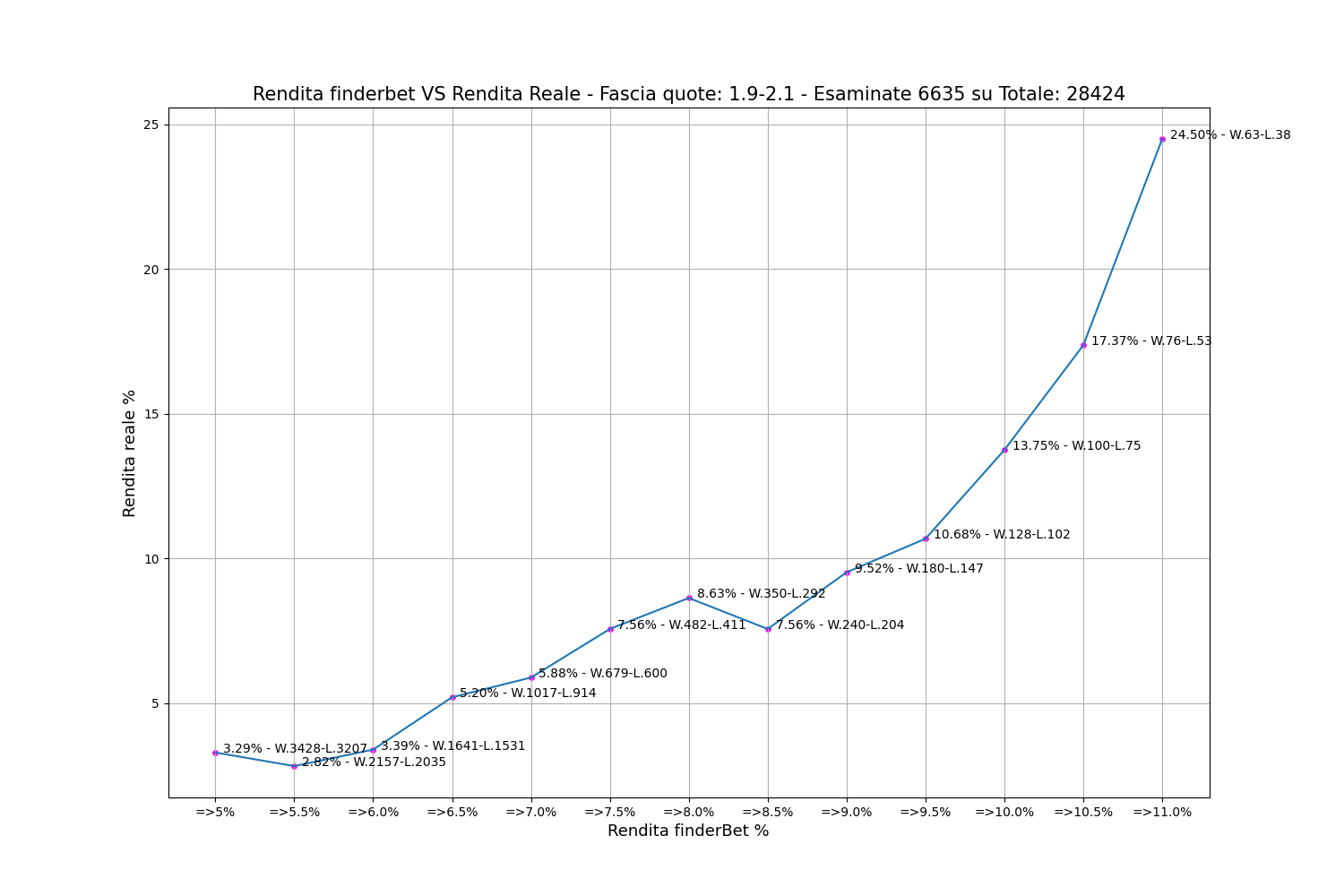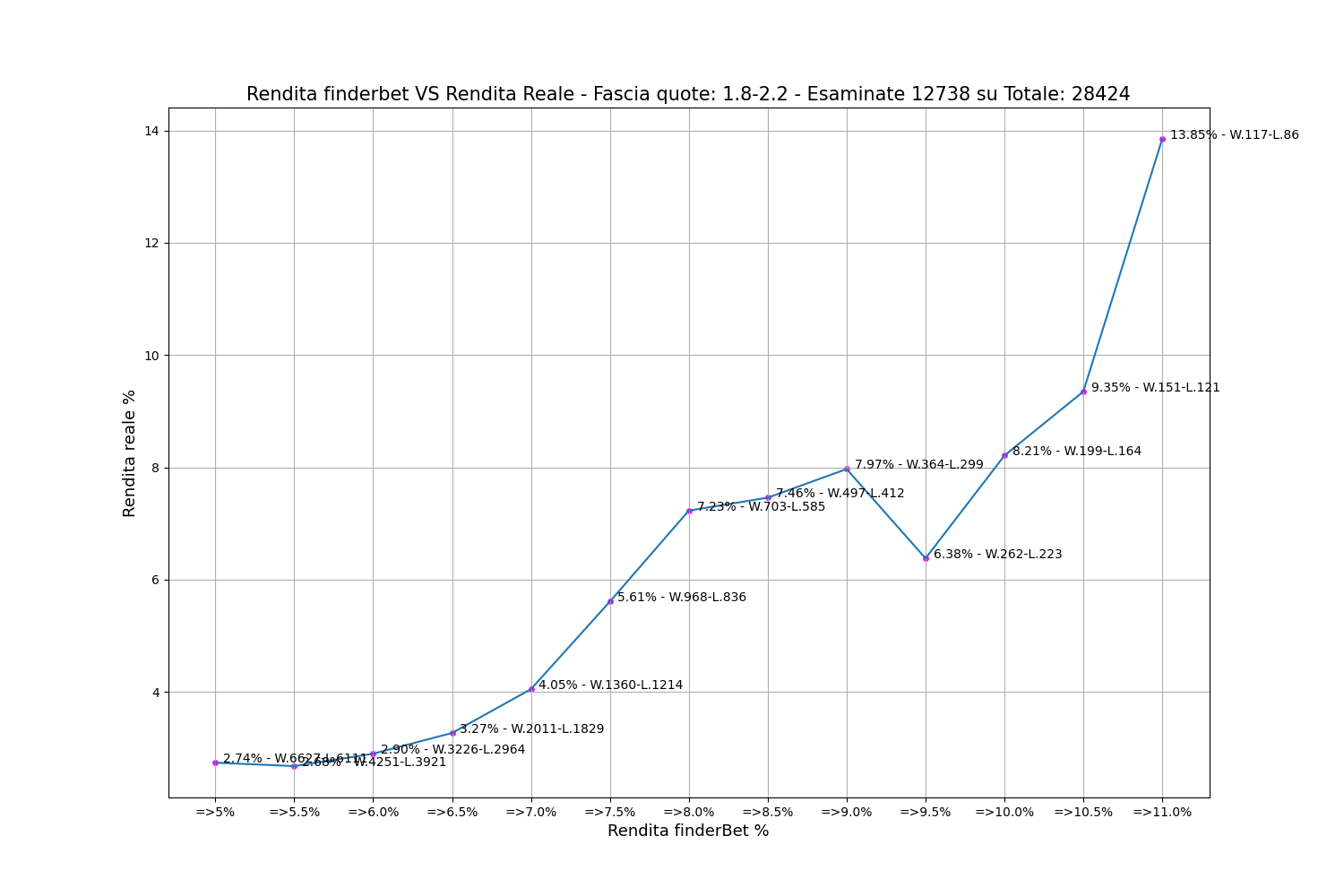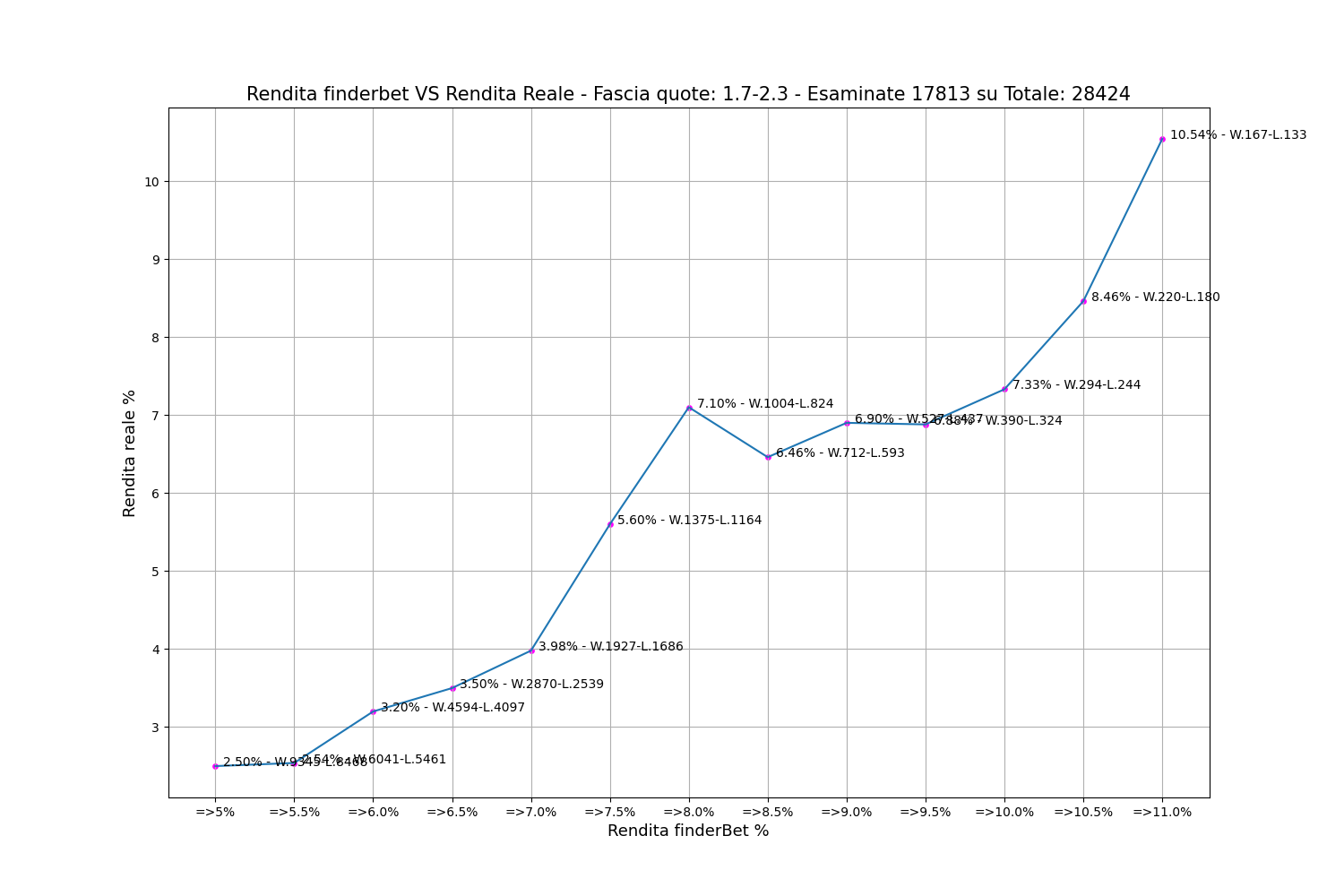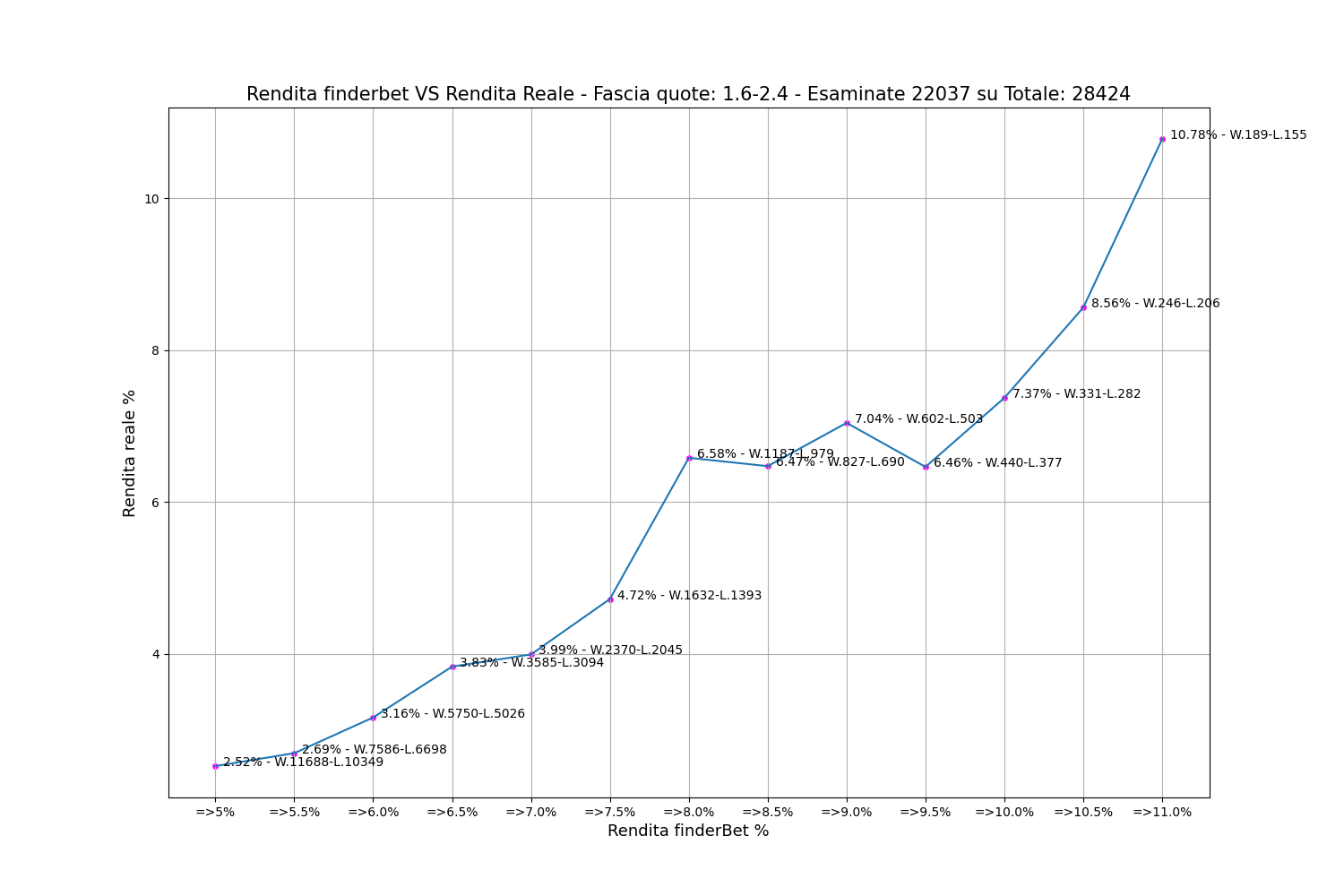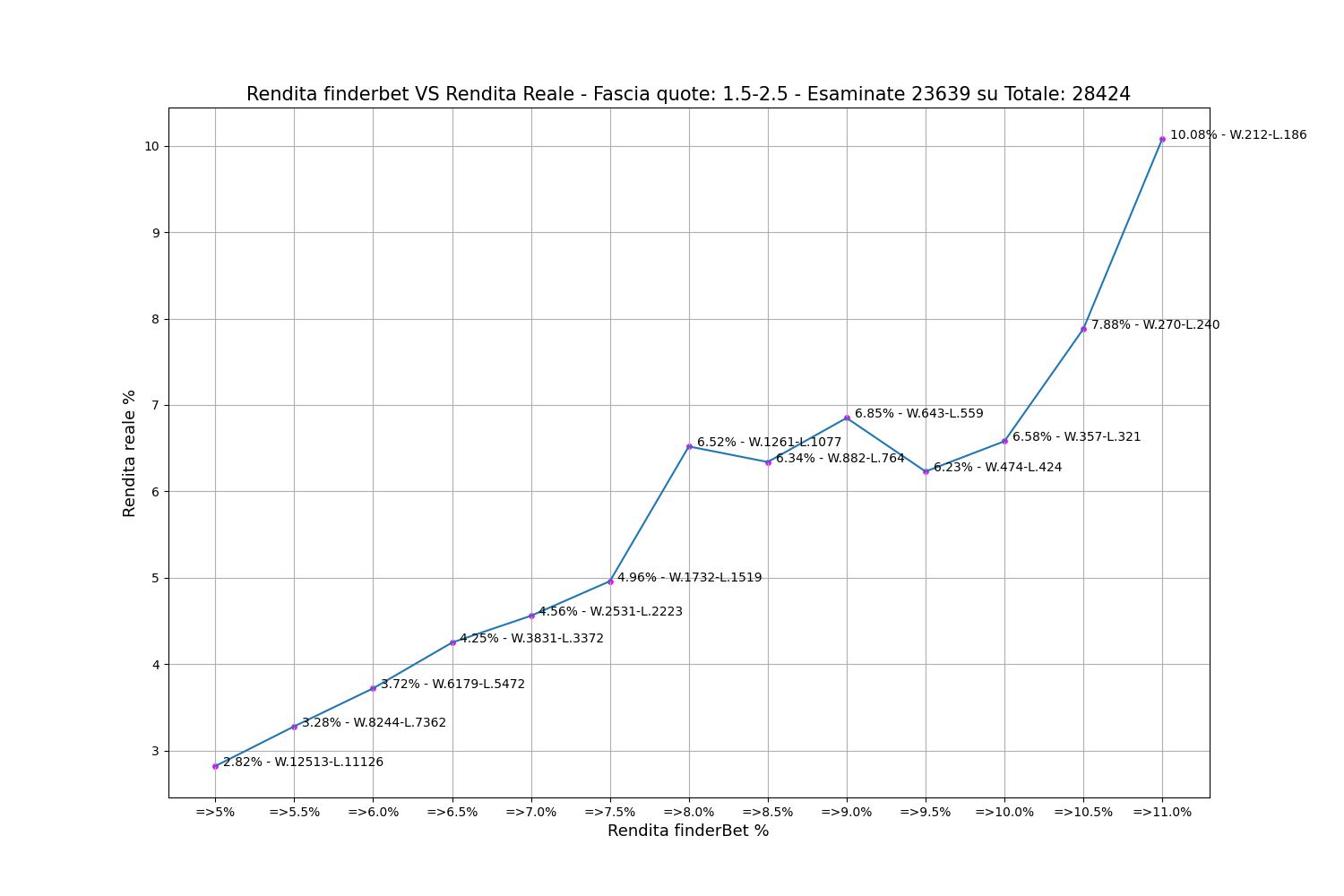### ValueBet: Statistics on individual books, January 2022 to present

Below is the data from the statistical study conducted on the real income of the ValueBet finderBet of the individual books, in documents that can be downloaded and consulted separately. If the graphs contained in the document do not reflect anything, it means that for that specific book there were no ValueBet “value odds”.

An example of the 5 graphs for each single book contained in the files:

ValueBet book – Odds range: 1.9-2.1 – Reviewed: X of Total: Y

ValueBet book – Odds range: 1.8-2.2 – Reviewed: X out of Total: Y

ValueBet book – Odds range: 1.7-2.3 – Reviewed: X out of Total: Y

ValueBet book – Odds range: 1.6-2.4 – Reviewed: X out of Total: Y

ValueBet book – Odds range: 1.5-2.5 – Reviewed: X out of Total: Y

With FinderBet you create your own constant income with

VALUERBET

Now you have more reasons to do so: